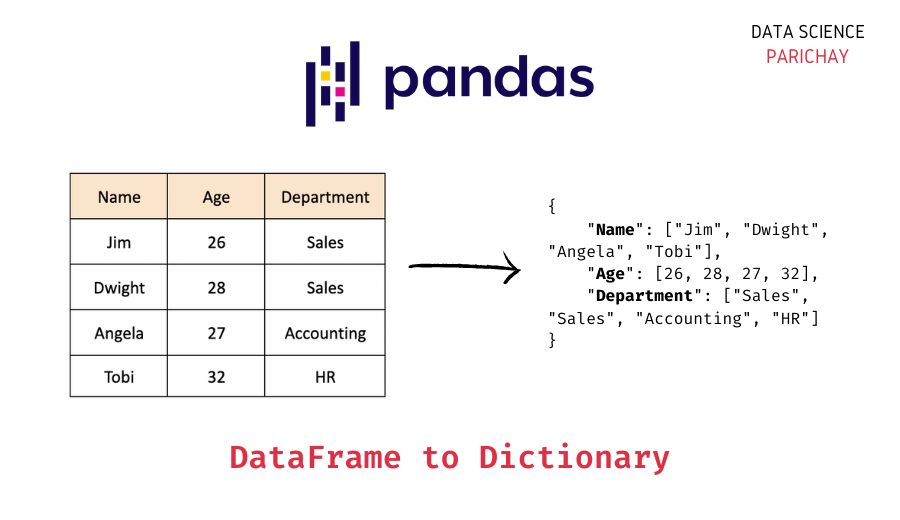# Convert Pandas DataFrame to a Dictionary

In this tutorial, we will look at how to convert a Dataframe in Pandas to a Python dictionary with the help of some examples.

## How to convert Pandas DataFrame to a Dictionary?

You can use the Pandas, `to_dict()` function to convert a Pandas dataframe to a dictionary in Python. The `to_dict()` function allows a range of orientations for the key-value pairs in the returned dictionary. The following is the syntax –

`d = df.to_dict(orient='dict')`

Here, `df` is the dataframe you want to convert. The `orient` parameter is used to determine the orientation of the returned dictionary. Its default value is `'dict'` which returns a dictionary in the form – `{column: {index: value}}`

Let’s look at the usage of the `to_dict()` function with the help of some examples.

First, we’ll create a sample dataframe that we’ll be using throughout this tutorial.

```# importing pprint to better print nested dictionaries
import pprint as pp
import pandas as pd

data = {
'Name': ['Microsoft Corporation', 'Google, LLC', 'Tesla, Inc.',\
'Apple Inc.', 'Netflix, Inc.'],
'Symbol': ['MSFT', 'GOOG', 'TSLA', 'AAPL', 'NFLX'],
'Shares': [100, 50, 150, 200, 80],
}

# create dataframe
df = pd.DataFrame(data, index=['Row1', 'Row2', 'Row3', 'Row4', 'Row5'])
# display the dataframe
df```

Output:

We now have a dataframe with information on a sample stock portfolio – the company name, stock symbol, and the shares count of the stocks in the portfolio.

📚 Data Science Programs By Skill Level

Introductory

Intermediate ⭐⭐⭐

🔎 Find Data Science Programs 👨‍💻 111,889 already enrolled

Disclaimer: Data Science Parichay is reader supported. When you purchase a course through a link on this site, we may earn a small commission at no additional cost to you. Earned commissions help support this website and its team of writers.

We also renamed the row indexes to better show (in subsequent examples) how the rows get represented in the dictionary returned by the the `to_dict()` function.

Now, let’s look at some of the different dictionary orientations that you use with the `to_dict()` function.

Using the pandas dataframe `to_dict()` function with the default parameter for `orient`, that is, `'dict'` returns a dictionary-like `{column: {index: value}}`. See the example below –

```# convert dataframe to dictionary
d = df.to_dict()
# print the dictionary
pp.pprint(d)```

Output:

```{'Name': {'Row1': 'Microsoft Corporation',
'Row3': 'Tesla, Inc.',
'Row4': 'Apple Inc.',
'Row5': 'Netflix, Inc.'},
'Shares': {'Row1': 100, 'Row2': 50, 'Row3': 150, 'Row4': 200, 'Row5': 80},
'Symbol': {'Row1': 'MSFT',
'Row2': 'GOOG',
'Row3': 'TSLA',
'Row4': 'AAPL',
'Row5': 'NFLX'}}```

In the above example, you can see the format of the dictionary returned. It has the column names as keys and the `{index: value}` mappings for that column as values.

If you want the returned dictionary to have the format `{column: [values]}`, pass `'list'` to the `orient` parameter.

```# convert dataframe to dictionary
d = df.to_dict(orient='list')
# print the dictionary
pp.pprint(d) ```

Output:

```{'Name': ['Microsoft Corporation',
'Tesla, Inc.',
'Apple Inc.',
'Netflix, Inc.'],
'Shares': [100, 50, 150, 200, 80],
'Symbol': ['MSFT', 'GOOG', 'TSLA', 'AAPL', 'NFLX']}```

You can see that the returned dictionary has the column names as keys and the list of column values as the respective value for each key.

If you want the returned dictionary to have the format `{column: Series(values)}`, pass `'series'` to the `orient` parameter.

```# convert dataframe to dictionary
d = df.to_dict(orient='series')
# print the dictionary
pp.pprint(d)
# check the type of the value
print("\nThe type of values:",type(d['Shares']))```

Output:

```{'Name': Row1    Microsoft Corporation
Row3              Tesla, Inc.
Row4               Apple Inc.
Row5            Netflix, Inc.
Name: Name, dtype: object,
'Shares': Row1    100
Row2     50
Row3    150
Row4    200
Row5     80
Name: Shares, dtype: int64,
'Symbol': Row1    MSFT
Row2    GOOG
Row3    TSLA
Row4    AAPL
Row5    NFLX
Name: Symbol, dtype: object}

The type of values: <class 'pandas.core.series.Series'>```

Here, the returned dictionary has the column names as keys and pandas series of the column values as the respective value for each key.

Now, instead of columns, if you want the returned dictionary to have the dataframe indexes as keys, pass `'index'` to the `orient` parameter. The returned dictionary has the format `{index: {column: value}}`

```# convert dataframe to dictionary
d = df.to_dict(orient='index')
# print the dictionary
pp.pprint(d)```

Output:

```{'Row1': {'Name': 'Microsoft Corporation', 'Shares': 100, 'Symbol': 'MSFT'},
'Row2': {'Name': 'Google, LLC', 'Shares': 50, 'Symbol': 'GOOG'},
'Row3': {'Name': 'Tesla, Inc.', 'Shares': 150, 'Symbol': 'TSLA'},
'Row4': {'Name': 'Apple Inc.', 'Shares': 200, 'Symbol': 'AAPL'},
'Row5': {'Name': 'Netflix, Inc.', 'Shares': 80, 'Symbol': 'NFLX'}}```

Here, you can see that the returned dictionary has row indexes as the keys and `{column: value}` mapping for that row as the respective dictionary value.

The `to_dict()` function also allows you to split your dataframe with the returned dictionary having the format `{'index': [index], 'columns': [columns], 'data': [values]}`. For this, pass `'split'` to the `orient` parameter.

```# convert dataframe to dictionary
d = df.to_dict(orient='split')
# print the dictionary
pp.pprint(d)```

Output:

```{'columns': ['Name', 'Symbol', 'Shares'],
'data': [['Microsoft Corporation', 'MSFT', 100],
['Tesla, Inc.', 'TSLA', 150],
['Apple Inc.', 'AAPL', 200],
['Netflix, Inc.', 'NFLX', 80]],
'index': ['Row1', 'Row2', 'Row3', 'Row4', 'Row5']}```

You can see that the returned dictionary is a result of splitting the dataframe into its individual components with the keys `'columns'`, `'data'`, and `'index'` and their respective values as lists.

For more on the pandas dataframe `to_dict()` function, refer to its official documentation.

With this, we come to the end of this tutorial. The code examples and results presented in this tutorial have been implemented in a Jupyter Notebook with a python (version 3.8.3) kernel having pandas version 1.0.5

•